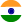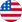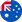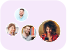24*7 support on WhatsAppChat NowProduct Edition:undefined
Author: Ian Naismith
Book Name: Elements of Partial Differential Equations
Subject Name: Maths

# Elements of Partial Differential Equations undefined Solutions

0 out of 5.0
51 reviews31 Students
have requested for homework help from this book

Geared toward students of applied rather than pure mathematics, this volume introduces elements of partial differential equations. Its focus is primarily upon finding solutions to particular equations rather than general theory. Topics include ordinary differential equations in more than two variables, partial differential equations of the first and second orders, Laplace's equation, the wave equation, and the diffusion equation. A helpful Appendix offers information on systems of surfaces, and solutions to the odd-numbered problems appear at the end of the book. Readers pursuing independent study will particularly appreciate the worked examples that appear throughout the text.

5
26
4
10
3
15
2
9
1
0

0

## Students who viewed this book also checked out